# Math in Focus Grade 3 Chapter 2 Practice 1 Answer Key Mental Subtraction

## Math in Focus Grade 3 Chapter 2 Practice 1 Answer Key Mental Subtraction

Example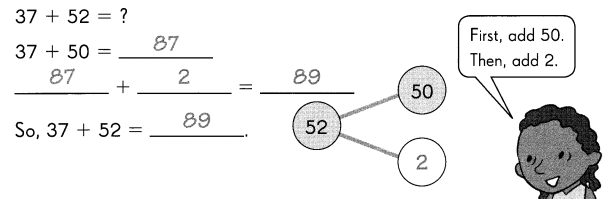Question 1.
24 + 55 = ?
24 + 50 = ___
___ + ___ = ___
So, 24 + 55 = ____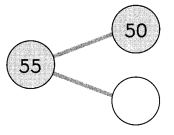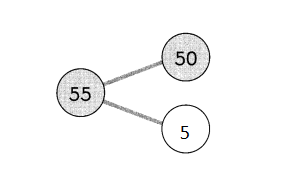24 + 50 = 74
74 + 5 = 79
So, 24 + 55 = 79

Question 2.
22 + 64 = ?
20 + 64 = ___
___ + ___ = ____
So, 22 + 64 = ___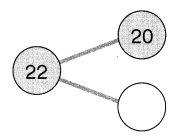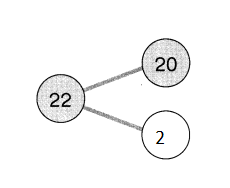20 + 64 = 84
84 + 2 = 86
So, 22 + 64 = 86

Example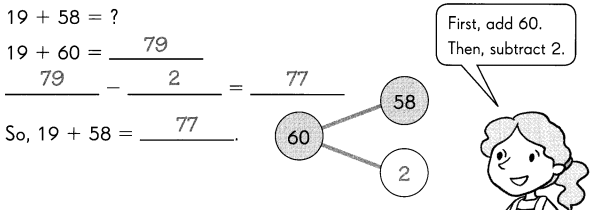Question 3.
37 + 45 = ?
37 + 50 = __
___ – ___ = ___
So, 37 + 45  = ___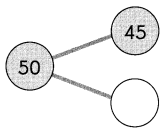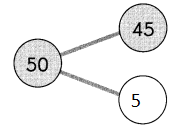37 + 50 = 87
87 – 5 = 82
So, 37 + 45  = 82

Question 4.
46 + 34 = ?
46 + 40 = ___
__ – __ = ___
So 46 + 34 = ___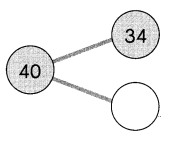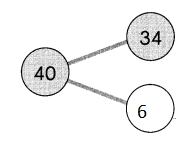46 + 40 = 86
86 – 6 = 80
So 46 + 34 = 80

Question 5.
41 + 43 = ___
41 + 43
40 + 40 = 80
80 + 1 + 3 = 84
So, 41 + 43 = 84

Question 6.
31 + 64 = ___
31 + 64
30 + 60 = 90
90 + 1 + 5 = 95
So, 31 + 64 = 95

Question 7.
15 + 47 = ___